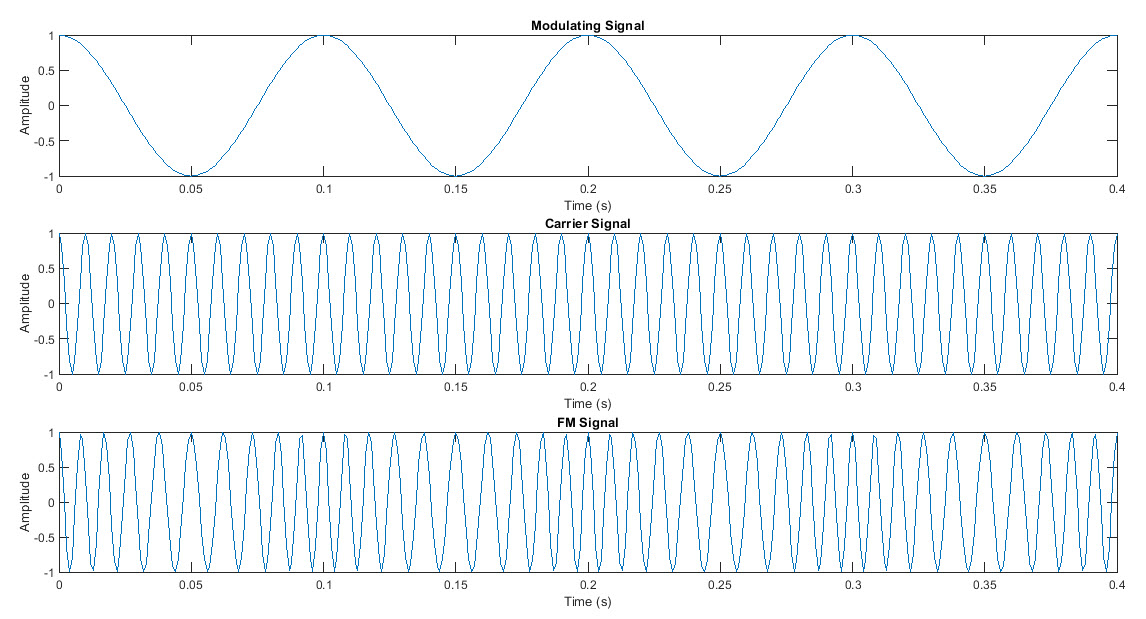# FM Modulation with MatlabAngle modulation is modulation technique wherein either the instantaneous phase or the frequency of carrier signal is varied according to the message signal. Thus we can classify angle modulation as Frequency Modulation and Phase Modulation.

An angle modulated signal is written mathematically as follows.

$$s(t) = A_c cos[\theta(t)]$$       ---------------->(1)

where the modulated signal amplitude $$A_c$$ is maintained constant and the angular argument $$\theta(t)$$ is varied according to the message signal $$m(t)$$.

The average frequency over an interval $$t$$ to $$\Delta t$$ is,

$$f_{\Delta t}(t) = \frac{\theta(t+\Delta t) - \theta(t)}{2 \pi \Delta t}$$

The instantaneous frequency is defined as the limiting value of average frequency as time goes to zero,

$$f_i(t) = \lim_{x\to \infty}f_{\Delta t}(t)$$

or,  $$f_i(t) = \lim_{x\to \infty}[\frac{\theta(t+\Delta t) - \theta(t)}{2 \pi \Delta t}]$$

or,  $$f_i(t) = \frac{1}{2 \pi} \frac{d \theta(t)}{dt}$$       -------------------->(2)

### Frequency Modulation

Frequency modulation is modulation process wherein the instantaneous frequency of a carrier signal is varied according to the message signal amplitude. In this modulation the modulated FM signal has changing frequency relating to the message amplitude but the amplitude of the FM signal remains constant. This form of FM modulation is analog modulation wherein the frequency of the carrier signal continuously changes with the message signal. FM modulation is used in FM transmitter for generating of FM signal.

Mathematically, the instantaneous frequency of the modulated FM signal is given by,

$$f_i = f_c + k_f m(t)$$         ---------------->(3)

where, $$f_c$$ is the unmodulated carrier signal frequency and $$k_f$$ is the frequency sensitivity of the FM modulator(Hz/V).

Integrating (2) w.r.t time and multiplying by $$2\pi$$,

$$\theta(t) = 2 \pi f_c t + 2 \pi k_f \int_{0}^{t}m(t) dt$$    ------------->(4)

From(1) the FM modulated signal is represented in time domain as follows,

$$s(t) = A_c cos[2 \pi f_c t + 2 \pi k_f \int_{0}^{t}m(t) dt]$$       ---------------->(5)

Let $$m(t)$$ be message signal which is given by,

$$m(t) = A_m cos(2 \pi f_m t)$$           ----------------->(6)

Substituting (6) into (5) we have,

$$s(t) = A_c cos[2 \pi f_c t + 2 \pi k_f \int_{0}^{t}A_m cos(2 \pi f_m t) dt]$$

or,  $$s(t) = A_c cos[2 \pi f_c t + 2 \pi k_f A_m \int_{0}^{t} cos(2 \pi f_m t) dt]$$   -------->(7)

Let, $$\Delta f = k_f A_m$$         ---------------->(8)

The quantity $$\Delta f$$ is called frequency deviation which is the maximum frequency deviation from the instantaneous frequency of the FM wave from the carrier frequency $$f_c$$. An FM signal has frequency deviation which is proportional to amplitude of message signal and is independent on the message frequency.

Using $$\Delta f$$ in equation(7),

$$s(t) = A_c cos[2 \pi f_c t + 2 \pi \Delta f \int_{0}^{t} cos(2 \pi f_m t) dt]$$

or, $$s(t) = A_c cos[2 \pi f_c t + 2 \pi \Delta f \frac{sin(2 \pi f_m t)}{2 \pi f_m } ]$$

or, $$s(t) = A_c cos[2 \pi f_c t + \frac{\Delta f }{f_m}sin(2 \pi f_m t)]$$

$$s(t) = A_c cos[2 \pi f_c t + \beta sin(2 \pi f_m t)]$$ ------------>(9)

### FM Modulation with Matlab

Matlab Code

The following is matlab code for generating FM signal.

Ac=input('enter carrier signal amplitude: ');
Am=input('enter message signal amplitude(Am<Ac): ');
fc=input('enter carrier frequency: ');
fm=input('enter message frequency(fm<fc): ');
beta=input('enter beta: ');

fs = 10*fc;   % Sampling frequency (Hz)
ts = 1/fs;    % Sampling period (s)
t = 0:ts:400*ts;   % Time vector (s)

% Generate modulating signal
m = Am*cos(2*pi*fm*t);

% Generate carrier signal
c = Ac*cos(2*pi*fc*t);

% Generate FM signal
f = cos(2*pi*fc*t + beta.*imag(hilbert(m)));

% Plot signals
subplot(3,1,1); plot(t,m); title('Modulating Signal');
subplot(3,1,2); plot(t,c); title('Carrier Signal');
subplot(3,1,3); plot(t,f); title('FM Signal');


This MATLAB code generates and plots a frequency modulated (FM) signal based on user-specified input parameters. The code prompts the user to enter the amplitude of the carrier signal (Ac), amplitude of the message signal (Am), carrier frequency (fc), message frequency (fm), and the modulation index (beta).

The code then sets the sampling frequency (fs) to 10 times the carrier frequency (fc) and calculates the sampling period (ts) based on the sampling frequency. It creates a time vector (t) that ranges from 0 to 400 times the sampling period (ts).

The modulating signal is generated using the message frequency (fm), the time vector (t), and the user-specified message signal amplitude (Am). The carrier signal is generated using the carrier frequency (fc), the time vector (t), and the user-specified carrier signal amplitude (Ac).

The FM signal is generated using the carrier frequency (fc), the time vector (t), the modulation index (beta), and the Hilbert transform of the modulating signal. The Hilbert transform is used to generate the analytic signal of the modulating signal, which is then multiplied by the modulation index (beta) and added to the carrier signal.

Finally, the code plots the modulating signal, carrier signal, and FM signal in separate subplots using the "subplot" command. This is shown below.

Overall, this code demonstrates how to generate an FM signal using MATLAB and provides an example of how to use the Hilbert transform to generate the analytic signal of a modulating signal.

Reference: